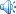# 2014年浙江教育出版社七年级下册数学暑假作业答案• 发布时间：2014-07-02 08:56:00
• 发布者：吾爱
• 微信号：wuaiyazhu.
• 浏览量：
• Tags：
•公告：

16、9003 17、 18、 -1 19、-6 20、99

（数轴正确0.5分，每一个点0.5分）
22、（1）17-8÷（-2）+3×（-7） （2）
=17+4-21 =
=0 =
= -7
23、解:
= (2分)
=

= 1+（-2）-1
= -2
24、解（1） 去括号：4x -3x+1 = 8 -6x

7x=7
X=1
（2）去分母 2(3a-1)=6-(4a-1)
6a-2 =6-4a+1
6a+4a =6+1+2
10a =9
a=0.9

25、（1） [5+0.7(x-3)]元 [或(0.7x+2.9)元]
（2） 当x=10时 0.7x+2.9 =0.7×10+2.9=9.9元

（3）3+（17.6-5）÷0.7=3+12.6÷0.7=21（千米）

5+0.7(x-3)=17.6
x=21